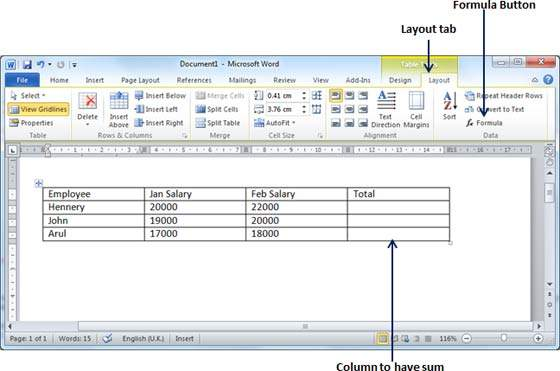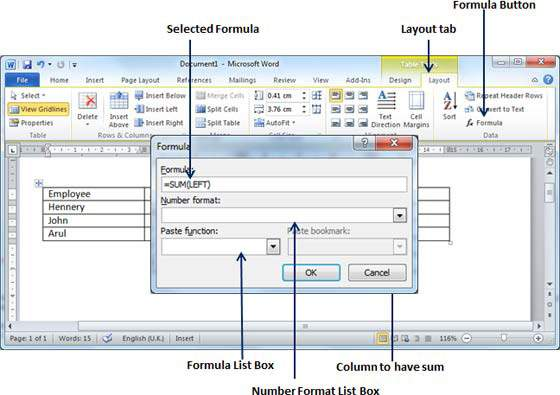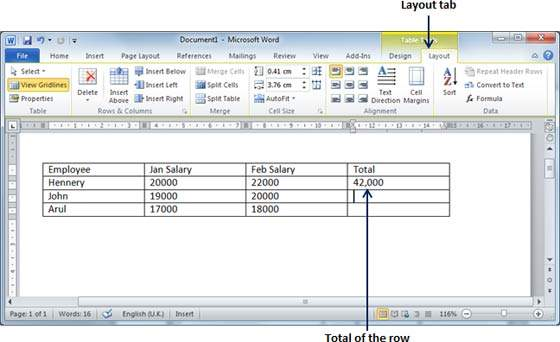# Add Formula in Word 2010

In this chapter, we will discuss how to add formula to a table in Word 2010. Microsoft Word allows you to use mathematical formula in table cells which can be used to add numbers, to find the average of numbers, or find the largest or the smallest number in table cells you specify. There is a list of formulae, you can choose from the many based on the requirement. This chapter will teach you how to use formula in word tables.

Following are the simple steps to add formula in a table cell available in Word document.

Step 1 − Consider the following table with the total number of rows. Click in a cell that should contain the sum of the rows.Step 2 − Now click the Layout tab and then click the Formula button; this will display a Formula Dialog Box which will suggest a default formula, which is =SUM(LEFT) in our case. You can select a number format using Number Format List Box to display the result or you can change the formula using the Formula List Box.Step 3 − Now click OK to apply the formula and you will see that the left cells have been added and the sum has been put in the total cell where we wanted to have it. You can repeat the procedure to have the sum of other two rows as well.## Cell Formulae

The Formula dialog box provides the following important functions to be used as formula in a cell.

S.No Formula & Description
1

AVERAGE( )

The average of a list of cells

2

COUNT( )

The number of items in a list of cells

3

MAX( )

The largest value in a list of cells

4

MIN( )

The smallest value in a list of cells

5

PRODUCT( )

The multiplication of a list of cells

6

SUM( )

The sum of a list of cells

We assume you are familiar with how to create a spreadsheet program; you can construct your word cell formula. Word formulae uses a reference system to refer to an individual table cells. Each column is identified by a letter, starting with A for the first column, B for the second column, and so on. After the letter comes the row number. Thus, the first cell in the first row is A1, the third cell in the fourth row is C4, and so on.

Following are useful points to help you in constructing a word cell formula.

S.No Cell References and Description
1 A single cell reference, such as B3 or F7
2 A range of cells, such as A4:A9 or C5:C13
3 A series of individual cells, such as A3, B4, C5
4 ABOVE, referring to all cells in the column above the current cell.
5 BELOW, referring to all cells in the column below the current cell.
6 LEFT, referring to all cells in the row to the left of the current cell
7 RIGHT, referring to all cells in the row to the right of the current cell

You can also construct simple Math expressions, such as B3+B5*10 by using simple mathematical operators +, -, /, *, %.